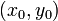# Peano existence theorem

## Statement

Consider a first-order differential equation in explicit form (note that any first-order first-degree differential equation can be converted to such a form):$\frac{dy}{dx} = G(x,y)$

Suppose$G$ is a continuous function (in a jointly continuous sense) on an open subset of$\R^2$ containing a point$(x_0,y_0)$. Then, there exists a function$f$ defined on an open subset$I$ of$\R$ containing$x_0$ such that$f$ satisfies the initial value problem, namely:$f(x_0) = y_0 \qquad \mbox{ and } f'(x) = G(x,f(x)) \ \forall \ x \in I$